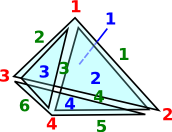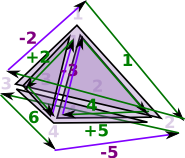# Delta Complexes Code

### Delta Complex

 When we looked at the delta complex we got a chain of 'face maps' between each dimension and the next lower one.In homology we treat this as a chain of abelian groups (more detail on the page here).

Sometimes, rather than defining faces, of any dimension, by their vertices It is useful to define each dimention in terms of the next lower dimenstion, for example, when generating homotopy groups such as the fundamental group.

To do this we represent the complex as a sequence of 'face maps' each one indexed into the next.

The ordering of the indexes is important. The indexes of any face are always ordered acording to their order in the next order face.

As an example consider the representation for a single tetrahedron:

The usual representation would be: (1,2,3,4)
which is very efficient but it does not allow us to refer to its edges and triangles.

Here we have indexed:

• The 4 vertices (in red)
• The 6 edges (in green)
• The 4 triangles (in blue)This representation holds all these indexes so that we don't have to keep creating them and they can be used consistently.

#### Face Maps

So the tetrahedron indexes its 4 triangles, each triangle indexes its 3 edges and each edge indexes its 2 vertices.

Each face table therefore indexes into the next. To show this I have drawn some of the arrows (I could not draw all the arrows as that would have made the diagram too messy).Since edges, triangles, tetrahedrons, etc. are oriented we need to put the indexed in a certain order.

 Edges are directed in the order of the vertex indices. That is low numbered index to high numbered index:For triangles we go in the order of the edges, except the middle edge is reversed. On the diagram the edges are coloured as follows: green arrows - not reversed purple arrows - reversed This gives the triangles a consistent windingHowever the whole tetrahedron is not yet consistent because some adjacent edges go in the same direction and some go in opposite directions.This gives the orientation of the faces as given by the right hand rule. That is: if the thumb of the right hand is outside the tetrahedion, pointing toward the face, then the fingers tend to curl round in the direction of the winding.The higher order faces may continue on in this manor, however it is difficult to visualize.

So to represent this structure we use the following:

`Rep := Record(VERTS:VS,SIMP:List(List(Integer)))`

where:

• VS is a list of verticies in whatever form we are using.
• Integer is an index, a negative sign reverses the direction.
• The inner list represents one of the tables shown above, edge has 2 entries, triangle has 3, and so on.
• The outer list holds all the tables, edge first, then triangles and so on.

### Example

 Here is an example of a tetrahedron (2-sphere).```(1) -> DELTA := DeltaComplex(VertexSetAbstract) (1) DeltaComplex(VertexSetAbstract) Type: Type (2) -> b4 := sphereSolid(3)\$SimplicialComplexFactory (2) [1 2 3 4] Type: FiniteSimplicialComplex(VertexSetAbstract) (3) -> c4 := deltaComplex(b4) (3) edge:[[1,2],[1,3],[1,4],[2,3],[2,4],[3,4]] triangle: [[1,2,4],[1,3,5],[2,3,6],[4,5,6]] tetrahedron: [[1,2,3,4]] Type: DeltaComplex(VertexSetAbstract)```